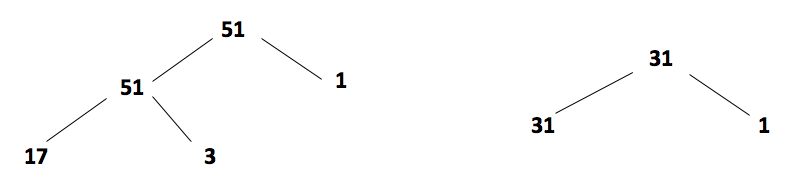What are prime numbers? A prime number is a number bigger than 1 which can only be divided evenly by 1 or itself.

For example, 13 is a prime number because it cannot be divided by any number except 13 or 1. Whereas 14 is not a prime number, as it can be divided by 2 and 7, as well as by 1 and itself.

These are the prime numbers under 20:

2,3,5,7,11,13,17 and 19.

With the exceptions of 0 and 1, any number which is not a prime number is called a composite number. A composite number is a whole number that can be divided evenly by numbers other than 1 or itself.

0 and 1 are not prime or composite numbers because they can only be divided by themselves.

## When do children learn about prime numbers?

Teachers will introduce children to prime numbers in Year 5 and by Year 6 children should be able to name the prime numbers up to 19.

Children will also be expected to be comfortable working out whether numbers up to 100 are prime or not. To practice their knowledge, children will be given questions like the following:

## The number 51 has 4 factors, which 2 of these factors are prime numbers?

Answer: 3 and 17. The other factors are 1 and 51.

This question requires children to also use their knowledge of factors, which they will be taught about at the same time as prime numbers.

## How to help children with prime numbers?

One way of helping children answer these kind of questions is by creating factor trees. Factor trees are especially useful when children are presented with bigger numbers, as they break down numbers into their prime factors. Here are examples of factor trees for the numbers 51 and 31:The factor tree for the number 51 shows that it is not a prime number but a composite number, as it can be calculated by multiplying either 1 x 51 or 17 x 3. This factor tree is also helpful to answer the question above, as it shows that 17 and 3 are both prime numbers. They cannot be divided by numbers other than 1 or themselves.

After getting children to work out which numbers multiply together to make 31, they will see that the only option is 1 x 31. So, the factor tree shows that 31 is a prime number, as it can only be divided by 1 and itself.

This exercise can really help children to consolidate their knowledge of factors, prime numbers and multiples.

## How does Learning Street help children with prime numbers?

Learning Street teaches introduces the concept of prime numbers to children and then gradually builds up their knowledge. This regular exposure and revision and development work ensures children make progress in a way that is often impossible just by using worksheet providers or books.

In fact, many tutors and tuition centres struggle to build up knowledge in such a complete way.

## Our Courses

Click through to review the courses we have available

##### Get Started

Learning Street structured courses make home study easier and more successful. There's no need to wonder which books to buy or what to do next. Everything is planned for you.

• Proven to deliver excellent results
• Used by families, private tutors and schools
• Fully planned and structured